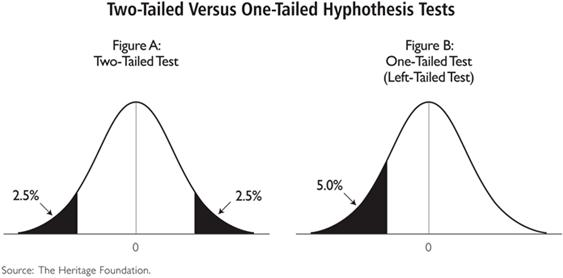# Conceptually understand Z-score obtained from tables

Hi,

I am trying to conceptually understand the following …

For a two-sided test … alpha = 5%, gives z-score = 1.96 (i.e. you have 95% probability between tails)

But for a one-sided tested … alpha = 5%, i.e., you have 97.5% probability between tails, why z-score be 1.65? I mean you have higher probability, so I don’t understand why z-score would be lower than that of a two-sided test.

Any help to understand this will be appreciated.

Nope.

You have 90% between the (two @ 5%) tails.